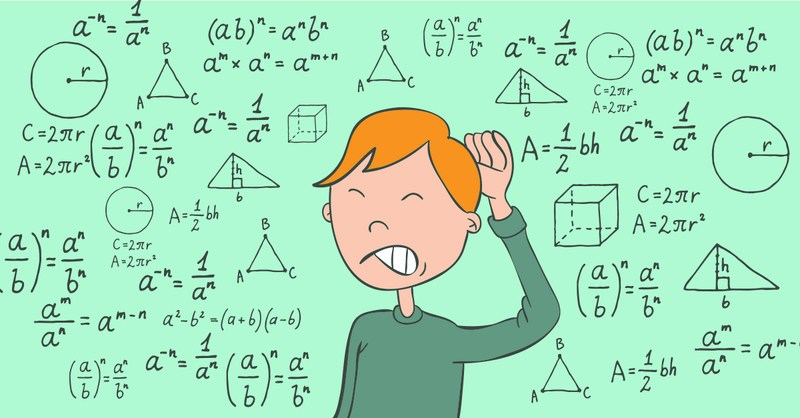# GMAT & MBA Admissions BlogThis post is the second in our series on using strategies to answer specific questions from the 2018 Official Guide. Here, one of our most experienced GMAT tutors, John Easter, analyzes a question about prime numbers using problem solving skills.

Problem #44 of the 2018 Official Guide to the GMAT states that if n is a prime number greater than 3, what is the remainder when n^2 is divided by 12?

(A) 0

(B) 1

(C) 2

(D) 3

(E) 5

I call these kinds of problems Theorem Problems. What I mean by that is that there is some deep mathematical rule that forms the basis of the problem. In this case that rule is about squares modulo p and quadratic residues. Neither of these is part of what the GMAT is testing - even if you're treating the quantitative section as a math test and not a game.

Clearly someone working for GMAC is nerding out. Unfortunately for the nerd in question, and fortunately for us, mathematicians don't do multiple choice. When you see something like this, take a deep breath and remember that PS (problems solving) questions are matching games. We just have to match the question to the answer. We don't need math degrees.

So, pick a prime greater than 3, square it, and divide by 12. What's the remainder?

For example, 5 is a prime greater than 3 (in fact, the smallest prime greater than three - keep it simple), 5^2 is 25, and when 25 is divided by 12, the remainder is 1. The correct answer is B. It's really that simple.

Now, an important question: Do we need to check another example? The answer is NO! There's only one answer per question!